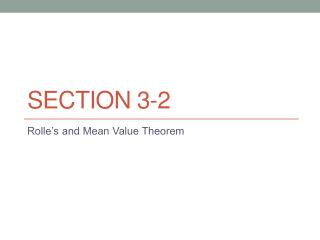DownloadDownload PresentationSection 3-2

# Section 3-2

Télécharger la présentation## Section 3-2

- - - - - - - - - - - - - - - - - - - - - - - - - - - E N D - - - - - - - - - - - - - - - - - - - - - - - - - - -
##### Presentation Transcript

1. Section 3-2 Rolle’s and Mean Value Theorem

2. a c b Rolle’s Theorem Let f be differentiable on (a,b) and continuous on [a,b]. If , then there is at least one point c belonging to (a,b) where

3. 1. Determine whether Rolle’s Theorem can be applied on the interval, then find the values of c in the interval given the function with an interval of [1,2]

4. 2.) Determine whether Rolle’s Theorem can be applied on the interval, then find the values of c in the interval given the function with an interval of [-2,2]

5. 3. Determine whether Rolle’s Theorem can be applied on the interval, then find the values of c in the interval given the function with an interval of [2,4]

6. c a b Mean Value Theorem • Let f be differentiable on (a,b) and continuous on [a,b], then there exists a point c belonging to (a,b) where

7. 4.) Find the number which satisfies the MVT for the function on [-1,3]

8. Find the number which satisfies the MVT for the function on [1,2]

9. 6.) Find the number which satisfies the MVT for the function on [0,p]

10. 7.) Suppose the police time you going from one mile to the next in 51.4 seconds. If you are traveling in a 55 mph zone, do you deserve a ticket?

11. Homework pg 176 # 2, 3, 11,12,14,15,18,20,23, 39,40, 42,43, 44, 46, and 47